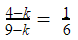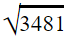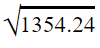# Number System TCS Questions For SSC 2021 in Hindi and English

Validity: 9 Months
What you will get
Course Highlights
• Based on latest Pattern
• English Medium eBooks
SSC

In this, we are providing previous year questions and solutions of SSC CGL CHSL CPO MTS of number system chapter asked by TCS. It is helpful for upcoming exams of SSC Railways and Other competitive exams conducted by TCS.

## Number System Chapter Practices Questions

Q1. If a/b=3/4, b/c=4/5, and c/d=56, then the sum of the numerator and the denominator (which are coprimes) of (a/d)10 is:

यदि a/b=3/4, b/c=4/5 और c/d=5/6 है, तो (ad)10 के अंश एवं हर ( जो सह-अभाज्य हैं ) का जोड़ क्या होगा?

SSC MTS 9 August

(a) 1025

(b) 4097

(c) 2049

(d) 513

Q2. A fraction is such that the numerator is five less than the denominator. Also four times the numerator is one more than the denominator. The fraction is:

एक भिन्न इस प्रकार है कि अंश , हर से 5 कम है | साथ ही, अंश का चार गुना हर से एक अधिक है | यह भिन्न है :

SSC MTS 9 August 2019 (Afternoon)

(a) 4/7

(b) 3/8

(c) 7/12

(d) 2/7

Q3. Which number should be subtracted from the numerator and denominator of the fraction 4/9so that the fraction could be made equal to 1/6

भिन्न 4/9 के अंश तथा हर प्रत्येक में कौन सी संख्या घटाई जानी चाहिए जिससे भिन्न को 1/6 के बराबर बनाया जा सके?

(a) 3

(b) 7

(c) 2

(d) 5

Q4. Three times a number is 24 more than the one-third of this number. This number is :

किसी संख्या का तीन गुना उसकी एक-तिहाई से 24 अधिक है | संख्या क्या है:

SSC MTS 21 August 2019 (Afternoon)

(a) 9

(b) 15

(c) 12

(d) 8

Q5. Let x be the greatest number which when divides 6475, 4984, and 4132, the remainder in each case is the same. What is the sum of digits of x?

मान लीजिये कि x सबसे बड़ी संख्या है जो 6475, 4984 और 4132 को विभाजित करने पर हर मामले में समान शेषफल छोड़ती है | x के अंकों का जोड़ क्या है ?

SSC MTS 22 August 2019 (Morning)? ???

(a) 4

(b) 7

(c) 5

(d) 6

Q6. When 6892, 7105, and 7531 are divided by the greatest number x, then the remainder in each case is y. What is the value of (x-y)?

जब 6892, 7105 और 7531 को सबसे बड़ी संख्या x से विभाजित किया जाता है, तो प्रत्येक मामले में शेषफल y आता है | (x-y) का मान ज्ञात करें |

SSC MTS 22 August 2019 (Afternoon)

(a) 123

(b) 137

(c) 147

(d) 113

Q7. x is the greatest number by which, when 2460, 2633, and 2806 are divided, the remainder in each case is the same. What is the sum of digits of x?

x वह सबसे बड़ी संख्या है जिससे 2460, 2633 तथा 2806 को विभाजित करने पर, हर मामले में शेषफल समान आता है | x के अंकों का योग क्या है ?

SSC MTS 22 August 2019 (Evening)

(a) 11

(b) 1

(c) 13

(d) 9

Q8. Given n is an integer, what is the remainder when (6n+3)²is divided by 9?

दिया गया n एक पूर्णांक है | जब (6n+3)2 को 9 से भाग दिया जाता है, तब शेषफल क्या आएगा?

SSC CGL 8 July 2019 (Afternoon)

(a) 3

(b) 2

(c) 1

(d) 0

Q9. The students of a class donated Rs. 3,481 towards the relief fund. Each student donated an amount equal to the number of students in the class. The number of students in the class is:

SSC CPO 16 March 2019 (Morning)

(a) 49

(b) 51

(c) 59

(d) 61

Q10. The square root of which of the following is a rational number?

SSC CPO 12 March 2019 (Morning)

(a) 1250.49

(b) 6250.49

(c) 1354.24

(d) 5768.28

### Here are the solutions to the above questions in the easiest way. It will help you to save your precious time on exams.

Sol 1.

Ans. (a)

a : b : c : d

3 : 4

4 : 5

5 : 6

⇒ a : b : c : d =  3 :  4 : 5 : 6

⇒ a/d= 3/6= 1/2

(a/d)10= (1/2)10= 1/1024

Required sum = 1024 + 1 = 1025

Sol 2.

Ans. (d)

Let the denominator = d

numerator = d-5

According to the question

4(d-5) = d+1

4d-20=d+1

d= 7

Desired fraction =7-5 /7= 2/7

Sol 3.

Ans (a)

Let the desired number = k

According to the question24-6k=9-k

⇒ k = 3

Sol 4.

Ans. (a)

Let the number = 3k

According to the question

9k-k = 24

⇒ k=3

Desired number = 3k = 3x3 = 9

Sol 5.

Ans. (d)

x will be the HCF of the differences of the three numbers.

6475-4984 = 1491

4984-4132 = 852

6475-4132 = 2343

1491 = 3 x 7 x 71

852 = 2 x 2 x 3 x 71

2343 = 3 x 11 x 71

So, HCF of 2343, 1491 and 852 = 3 x 71 = 213

Sol 6.

Ans. (b)

If a number 'a' and a number 'b' are divisible by a number 'n' then, a+b and a-b is also divisible by n.

Here the numbers are 7531, 7105 and 6892

the required number then becomes H.C.F of 7531-y, 6892-y, and 7105-y or the HCF of (7531-y)-(7105-y) and (7105-y)-(6892-y)

(7531-y)-(7105-y) = 426

(7105-y)-(6892-y) = 213

213 = 3 x 71

426 = 2 x 3 x 71

So, HCF of 426 and 213 =x= 3 x 71 = 213

The remainder = 7531/213 = 7105/213 = 6892/213 = y = 76

⇒ (x-y)=213-76=137

Sol 7.

Ans. (a)

If a number 'a' and a number 'b' are divisible by a number 'n' then, a+b and a-b are also divisible by n.

Here the numbers are 2460, 2633, and 2806, and let the remainder = y

the required number then becomes H.C.F of 2460-y, 2633-y, and 2806-y or the HCF of (2633-y)-(2460-y) and (2806-y)-(2633-y)

(2633-y)-(2460-y) = 173

(2806-y)-(2633-y) = 173

So, HCF of 173 and 173 =x= 173

Desired sum = 1+7+3 = 11

Sol 8.

Ans. (d)

Given, (6n+3)²

On expanding the given polynomial, we get 36+36n+9

Since 36 and 9 are divisible by 9.

Therefore, On dividing (6n+3)² by 9. We get 0 as remainder.

Alternate :

Put any integer value of n for example 1.

(6n+3)²⇒{6(1)+3}²= 81

Since 81 is divisible by 9 the remainder will be 0.

Sol 9.

Ans. (c)

Let the number of students = s, so amount donated by each student = s

According to the question

s × s= 3481

⇒ s==59

Sol 10. (c) Since=36.8